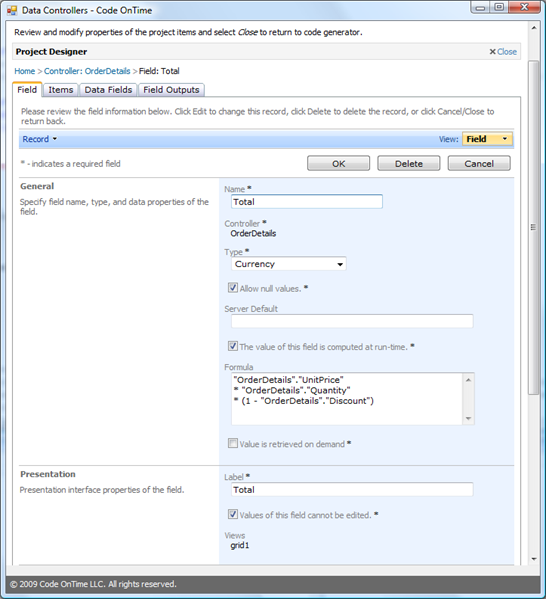# Calculated Fields Revisited

Labels
Archive
Blog
Friday, October 2, 2009PrintSubscribe
Calculated Fields Revisited

The upcoming update of Data Aquarium Framework will include additional support for field formulas to simplify creation of calculated fields.

The current available options are listed here:
/blog/2009/05/calculated-fields-in-data-controllers.html

The update will allow for a more flexible and much simpler solution. Here is an example of an actual calculated formula for OrderDetails.xml data controller:

```<field name="Total" type="Currency" allowNulls="true" isPrimaryKey="false" readOnly="true" label="Total" computed="true">
<formula>"OrderDetails"."UnitPrice" * "OrderDetails"."Quantity" * (1 - "OrderDetails"."Discount")</formula>
</field>
```

The “computed” attribute will enable the formula. The formula is an SQL expression that is embedded into final SELECT statement. You can create formulas for standard generated fields and quickly introduce new fields without the need to modify the original command or creating views.

Code OnTime Designer will fully support field formulas.